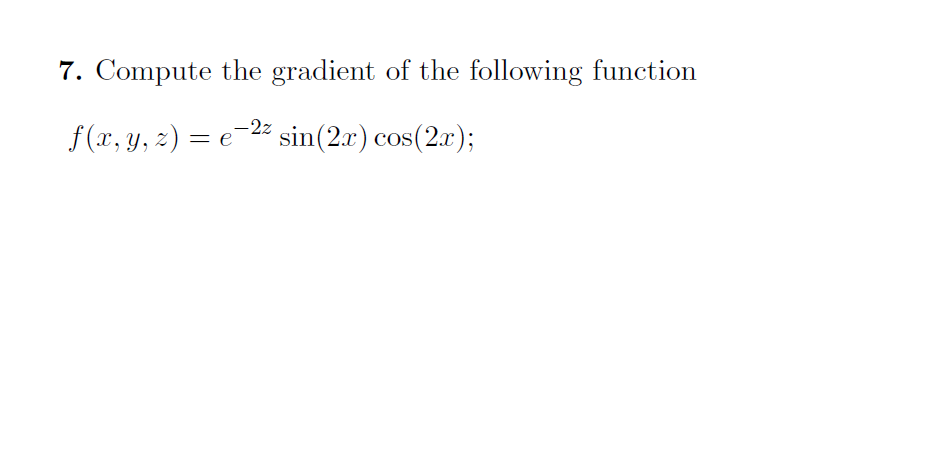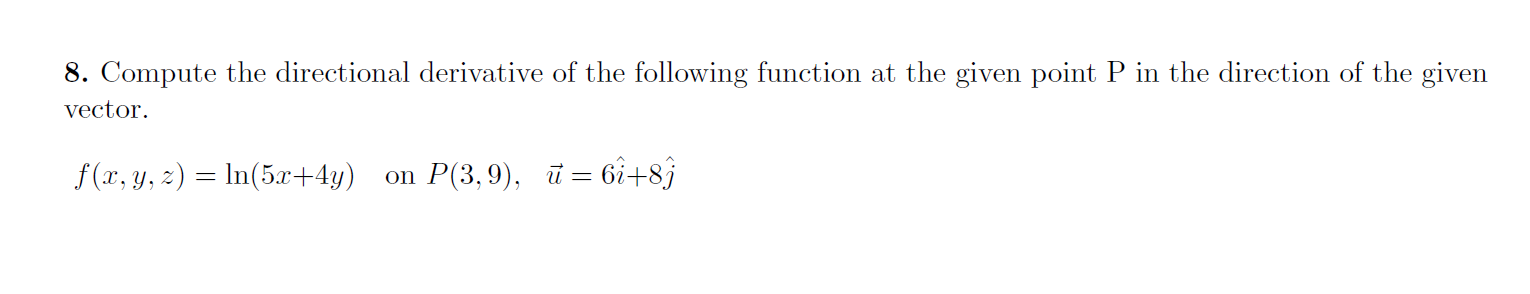### Create an Account

Already have account?

### Forgot Your Password ?

Home / Questions / 7. Compute the gradient of the following function f(x, y, z) = e-22 sin(2x) cos(2x); 8. Co...

# 7. Compute the gradient of the following function f(x, y, z) = e-22 sin(2x) cos(2x); 8. Compute the directional derivative of the following function at the given point P in the direction of the given

7. Compute the gradient of the following function f(x, y, z) = e-22 sin(2x) cos(2x);
8. Compute the directional derivative of the following function at the given point P in the direction of the given vector. f(x, y, z) = ln(5x+4y) on P(3,9), ū= 6i+89Apr 13 2021 View more View Less

#### Answer (Solved)Subscribe To Get Solution